Next: Discrete Hidden Variables CEM Up: CEM - A Maximum Previous: CEM - A Maximum

# From EM to CEM

In this chapter, we discuss the extension of the EM (Expectation Maximization) algorithm for joint density estimation to conditional density estimation and derive the resulting CEM (Conditional Expectation Maximization) algorithm. The machine learning system that is central to the Action-Reaction Learning paradigm requires conditional densities to model interaction. Thus, the optimization techniques described in the preceding chapter will be called upon to formally derive the necessary algorithm. The use of CEM is demonstrated for a specific probabilistic model, a conditioned mixture of Gaussians, and implementation and machine learning issues are discussed.

In joint density estimation, the tried and true EM (expectation maximization) algorithm proceeds by maximizing likelihood over a training set. By introducing the concept of missing data, the algorithm breaks down what would be a complex density optimization into a two-step iteration. The missing unknown data components are estimated via the E-step and then a far simpler maximization over the complete data, the M-step, is performed. This is in fact identical to the iteration described earlier for General Bound Maximization. The E-step basically computes a bound (via Jensen's inequality) for the likelihood and the M-step maximizes that bound. Iteration of this process is guaranteed to converge to a local maximum of likelihood.

Recall the maximum likelihood joint density estimation (ML) case in Equation 5.2. Imagine we are faced with such a joint density optimization task and need to compute an optimal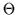over sets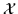and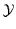of N points as in Equation 7.1.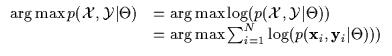(7.1)

The EM algorithm is an iterative optimization which begins seeded at an initial starting point in the model space (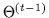). Instead of maximizing the expression in Equation 7.1, one can instead maximize its iterative form in Equation 7.2 where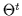is the algorithm's estimate for a better model from the previous estimate.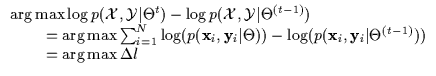(7.2)

The density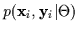in general is not simple and is often better described by the discrete or continuous summation of simpler models. This is always true when data is missing. In other words, it is a mixture model as in Equation 7.3. The summation is performed over what is typically called the 'missing components' (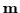or m) which are individually more tractable. Thus, the original rather complex pdf can be considered to be a linear integration or summation of simpler pdf models.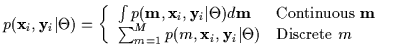(7.3)Next: Discrete Hidden Variables CEM Up: CEM - A Maximum Previous: CEM - A Maximum
Tony Jebara
1999-09-15